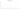# \$

## Bahamian dollar

Since 1966, The Bahamas have used the dollar as their official currency. In order to distinguish it from other currencies denominated in dollars, it is typically abbreviated with the dollar symbol \$ or, alternatively, B\$. There are 100 pennies in it. As an alternative to the traditional Bahamian dollar, the Bahamas introduced the Sand Dollar on October 20, 2020, making them the first nation to have a legal digital currency.

#### how has the value of the currency changed in the last year?## USD 1 = BSD 1

How much is10 US dollars worth inBahamian dollars?
At the current exchange rate, 10 US dollars is worth 10 Bahamian dollars
How much is50 US dollars worth inBahamian dollars?
At the current exchange rate, 50 US dollars is worth 50 Bahamian dollars
How much is100 US dollars worth inBahamian dollars?
At the current exchange rate, 100 US dollars is worth 100 Bahamian dollars
How much is500 US dollars worth inBahamian dollars?
At the current exchange rate, 500 US dollars is worth 500 Bahamian dollars
How much is2,000 US dollars worth inBahamian dollars?
At the current exchange rate, 2,000 US dollars is worth 2,000 Bahamian dollars
How much is10 US dollars worth inBahamian dollars?
At the current exchange rate, 10 US dollars is worth 10 Bahamian dollars
How much is50 US dollars worth inBahamian dollars?
At the current exchange rate, 50 US dollars is worth 50 Bahamian dollars
How much is100 US dollars worth inBahamian dollars?
At the current exchange rate, 100 US dollars is worth 100 Bahamian dollars
How much is500 US dollars worth inBahamian dollars?
At the current exchange rate, 500 US dollars is worth 500 Bahamian dollars
How much is2,000 US dollars worth inBahamian dollars?
At the current exchange rate, 2,000 US dollars is worth 2,000 Bahamian dollars